## Intoduction

This tutorial demonstrates the application of energyRt package to develop a Reference Energy System (RES, or Energy system optimization) model and conduct standard analysis, i.e. run scenarios, optimize several alternative development pathways of the simplified energy system. The initial (base-year) structure of the discussed below example of RES is flexible. The following features can be easily adjusted:
* number of regions and the model GIS-info (the map),
* the model horizon and annual time-steps (“milestone years”),
* number and levels of sub-annual time-steps (“time slices”),
* technological options, commodities, storage, supply, and demand,
* interregional trade and trade with the rest of the world (ROW),
* constraints on the model variables.

The energyRt package provides a set of S4 classes, methods, and functions to design the model elements, such as technologies, commodities, supply, demand, and constraints, save them in a model object, process the data and save it in a format readable by solver-software (GAMS, GLPK/Mathprog, Python/Pyomo, or Julia/JuMP), run the model code in the solver-software, read the results back to R, and manipulate the data to produce charts and tables.

This Vignette tutorial can be acquired from the energyRt/vignetts folder (or https://github.com/energyRt/energyRt/vignettes). It can be run step-by-step or at once to reproduce the results below. Playing with parameters and data is highly recommended for learning the package and the RES-models. This is the first beta-version of the package and the tutorial. Please report bugs, issues, thoughts here: https://github.com/energyRt/energyRt/issues.

## Prerequisites

We assume that R (https://www.r-project.org/) and RStudio (https://www.rstudio.com/) has been already installed, and a scholar has some basic knowledge of R. The next step is to have installed energyRt, the solver software (GAMS or GLPK) and LaTeX. The detailed installation steps are available on the package website (https://github.com/energyRt/energyRt/).

# Choose your solver-software
mysolver <- "GLPK"
# mysolver = "JuMP"
mysolver <- "Pyomo"
mysolver <- "GAMS"

if (T) {
# Installation of required packages - if not installed.
mypks <- rownames(installed.packages())
# General tools, data handling, visualisation
if (!any(mypks == "devtools")) install.packages("devtools")
if (!any(mypks == "tidyverse")) install.packages("tidyverse")
if (!any(mypks == "lubridate")) install.packages("lubridate")
# energyRt
if (!any(mypks == "energyRt")) devtools::install_github("olugovoy/energyRt")
# GIS tools
if (!any(mypks == "rgeos")) install.packages("rgeos")
if (!any(mypks == "rgdal")) install.packages("rgdal")
# if (!any(mypks == "gpclib")) install.packages("gpclib")
if (!any(mypks == "spdep")) install.packages("spdep")
if (!any(mypks == "maptools")) install.packages("maptools")
# Reporting
if (!any(mypks == "scales")) install.packages("scales")
if (!any(mypks == "kableExtra")) install.packages("kableExtra")
}
library(energyRt)
library(scales)
library(tidyverse)
library(lubridate)
library(data.table)

# Set color palette for figures
# palette(RColorBrewer::brewer.pal(11, "Paired"))
palette(RColorBrewer::brewer.pal(11, "Set3"))

## Utopia map

Let’s start with a multi-region map for the model. Below we use GIS info of this imaginary country for visualization of results and also to calculate some parameters for renewables, like solar radiation, and distances between regions. Several options of 11-region map with arbitrary GIS info is saved in energyRt/data folder and compared on the figure below.

### Available options

par(mfrow = c(2, 2), mar = seq(0.1, 4))
maps <- c("utopia_island", "utopia_continent", "utopia_squares", "utopia_honeycomb")
for (i in 1:4) {
gis <- get(data(list = maps[i])) # load map
sp::plot(gis, col = 1:length(gis), main = maps[i], bg = "aliceblue")
cn <- get_labpt_spdf(gis) # get coordinates of the regions' centers
text(cn$x, cn$y)
}Map options for Utopia models, up to 11 regions.

rm(gis, list = ls(pattern = "utopia_")) # clean-up
dev.off() # reset graphical parameters

Certainly, any other map in SpatialPolygonsDataFrame (spdf) format and with saved names of regions in @data$region column of the spdf object can be used instead. For this particular example lets pick utopia_honeycomb map and keep the 7 first regions for the simulation. data("utopia_honeycomb") # , package = "energyRt") gis <- utopia_honeycomb rm(utopia_honeycomb) gis <- gis[1:7, ] # gis <- gis[-c(8:9),] # Often used parameters (reg_names <- as.character(gis@data$region)) # Region names
(nreg <- length(reg_names)) # Number of regions
reg_centers <- getCenters(gis) # Coordinates of the regions' centers
(nmon <- length(timeslices$MONTH)) (nhou <- nslc / nmon) We will assign the time slices to the model-object later, but may also need the structure and/or names of the slices in the definition of other objects, such as technologies, demand, and supply, therefore useful to have them ready. ## Electric power sector Electric generation is likely the most often modeled sector of RES. It has a number of alternative technological options which can be evaluated and compared with RES-models. The minimum set for the model is a declaration of commodities, technologies, supply, demand, and basic system parameters, like time-slices, as discussed above. ### Commodities A “commodity” notion in the model is a generalization of goods, services, or emissions. Commodities link processes such as supply, technologies, demands as input or output. The minimum requirements to declare commodity is its name. Additional parameters can be stored in the class commodity for information and processing, most of the slots are currently reserved to be used in user-defined functions. The preferred way to create the class and fill it with data is the newCommodity() function as shown below. COA <- newCommodity( name = "COA", # the name as it appears in the solver-software and the model sets description = "Generic coal", # just a comment emis = list( # emissions, associated with fuels combustion (see the flags in technologies) comm = "CO2", # this commodity (CO2) is emmited when the fuel (COA) is used unit = "kt/PJ", # the unit of emissions for refference emis = 100 # i.e. 100 kt of CO2 emmitted per one unit of energy (1 PJ) ), slice = "ANNUAL" # 'ANNUAL' means no sub-annual granularity for the commodity # color = "brown" # reserved for output figures, optional ) OIL <- newCommodity( name = "OIL", description = "Oil and oil products", emis = list( comm = "CO2", emis = 80 ), slice = "ANNUAL" ) GAS <- newCommodity( name = "GAS", description = "Natural gas", emis = list( comm = "CO2", unit = "kt/PJ", emis = 70 ), slice = "MONTH" # This commodity will appear in month-level equations ) CH4 <- newCommodity( name = "CH4", description = "Methan emmisions", slice = "HOUR" ) BIO <- newCommodity( name = "BIO", description = "Generic biomass, all types", slice = "ANNUAL" ) # More energy commodities with less details ELC <- newCommodity("ELC", description = "Electricity", slice = "HOUR") HVE <- newCommodity("HVE", description = "High voltage electricity", slice = "HOUR") UHV <- newCommodity("UHV", description = "Ultra high voltage electricity", slice = "HOUR") NUC <- newCommodity("NUC", description = "Nuclear fuel", slice = "ANNUAL") HYD <- newCommodity("HYD", description = "Hydro energy", slice = "HOUR") SOL <- newCommodity("SOL", description = "Solar energy", slice = "HOUR") WIN <- newCommodity("WIN", description = "Wind energy", slice = "HOUR") # Emissions CO2 <- newCommodity("CO2", description = "Carbon dioxide emissions", slice = "HOUR") NOX <- newCommodity("NOX", description = "Nitrogen oxide emissions", slice = "HOUR") SO2 <- newCommodity("SO2", description = "Sulfur dioxide emissions", slice = "HOUR") HG <- newCommodity("HG", description = "Mercury emissions", slice = "HOUR") PM <- newCommodity("PM", description = "Particulate matter emissions", slice = "HOUR") ### The final demand The final product in this model will be electricity (ELC), the final demand for ELC is exogenous. Since we defined ELC as an hour-level commodity, we specify demand for every time slice (the load curve) and every region where it is consumed. The number of hours (or groups of hours) is equal to the time-slices. In the case of the ### Demand by months and hours hours Now let’s specify annual, monthly, and hourly demand for electricity, assuming that every region has a different level of ELC consumptions and the loadcurve. Instead of googling and web-scrapping the Utopia’s load curve, we generate it randomly for our example. # Annual demand in 2015 elc_dem_a <- tibble( region = reg_names, year = 2015, GWh = seq(5000, by = 500, length.out = nreg) ) elc_dem_a fLoadCurve <- function(n = 24, seed = NULL, delt = 24 / n / 20) { # a function to generate an arbitrary load curve if (!is.null(seed)) set.seed(seed) iter <- TRUE lc <- 1. for (i in 2:n) { # ARMA lc[i] <- 1 * lc[i - 1] + -.1 * (lc[i - 1] - 1) + runif(1, -delt, delt) } (0 + lc) * n / sum(lc + 0) } # Hourly demand for every region set.seed(1) dem <- tibble( region = rep(reg_names, each = nslc), year = 2015, slice = rep(slc$slice, nreg),
GWh = c(sapply(elc_dem_a$GWh, function(x) { (x * fLoadCurve(nmon)) %x% fLoadCurve(nhou) })) / nslc ) head(dem) sum(dem$GWh)

# Check by aggregating back to annual numbers
dem %>%
group_by(region) %>%
summarise(GWh_agg = sum(GWh)) %>%
mutate(GWh_a = elc_dem_a$GWh) # Add month and hour for plots # dem$month <- month.abb[as.numeric(substr(dem$slice, 2, 3))] # dem$hour <- as.integer(substr(dem$slice, 6, 7)) dem <- left_join(dem, select(slc, MONTH, HOUR, slice, share), by = "slice") %>% rename(month = MONTH) dem$hour <- as.numeric(as.factor(dem$HOUR)) ggplot(dem, aes(hour, GWh)) + geom_line(aes(color = region)) + ylim(c(0, NA)) + facet_wrap(. ~ month) + theme_bw() + labs(title = "(Assumed) Electricity consumption by hours in the base year") + theme(plot.title = element_text(hjust = 0.5))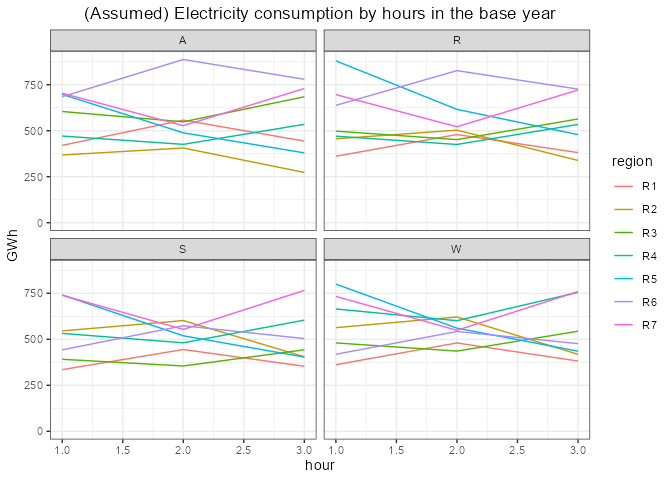The demand for 2015 is done, now we need to provide projections of the demand up to the last year of the model horizon, and with the same level of granularity (regions * slices) as the base year, and the commodity (ELC) itself. We can specify the demand for every (milestone) year of the model horizon, or some of them. The values between specified years will be interpolated If the model horizon goes beyond the last year, the final values will be used for interpolation forward. # Adding annual demand for 2030 and 2055 elc_dem_2030 <- dem elc_dem_2030$year <- 2030
elc_dem_2030$GWh <- dem$GWh * 2 # assuming two-fold growth by 2030

elc_dem_2055 <- dem
elc_dem_2055$year <- 2055 # The model horizon elc_dem_2055$GWh <- dem$GWh * 3 # and 3-times by 2055 elc_dem <- dem %>% bind_rows(elc_dem_2030) %>% bind_rows(elc_dem_2055) # The model units in PJ, converting... elc_dem$PJ <- convert("GWh", "PJ", elc_dem$GWh) elc_dem$region <- as.character(elc_dem$region) DEM_ELC <- newDemand( name = "DEM_ELC", description = "Final demand for electricity", commodity = "ELC", dem = list( year = elc_dem$year,
region = elc_dem$region, slice = elc_dem$slice,
dem = elc_dem$PJ ) ) dim(DEM_ELC@dem) unique(DEM_ELC@dem$year)
length(unique(DEM_ELC@dem$region)) length(unique(DEM_ELC@dem$slice))

### Primary supply

Primary commodities, such as primary energy sources, materials, appear in the RES-model either by export from the rest of the world, or supply. Neither, supply or export from ROW has capacity per se. The main difference between them is the origin of the commodity if it is domestic or foreign Here we assume that different regions of Utopia have different resources to stimulate trade between them. Function newSupply creates supply object with declared parameters. The annual, regional, or slice-level limits, as well as costs, can be assigned with availability parameters.

# If we assume that coal is abundunt resource, available in every region with the same price,
# we don't have to declare @region of the supply.
SUP_COA <- newSupply(
name = "SUP_COA",
description = "Supply of coal",
commodity = "COA",
unit = "PJ",
reserve = list(res.up = 1e6), # total limit of the resource (i.e. deposits)
region = reg_names,
availability = list(
year = c(2015, 2030, 2050),
ava.up = c(1000, 2000, 1000), # the upper bound on availability of the commodity
cost = c(convert("USD/tce", "MUSD/PJ", c(50, 60, 70) * .7)) # assumed price per 1PJ
),
slice = "ANNUAL"
)

The saved parameter can be checked in SUP_COA@availability slot:

region year slice ava.lo ava.up ava.fx cost
NA 2015 NA NA 1000 NA 1.194213
NA 2030 NA NA 2000 NA 1.433056
NA 2050 NA NA 1000 NA 1.671898

The NA values mean “for all” by default in columns with sets (region, year, slice) or “no constraint” in columns with numeric parameters. The values between the specified years will be linearly interpolated by default.
Similarly, for other primary commodities:

set.seed(100)
SUP_GAS <- newSupply(
name = "SUP_GAS",
description = "Supply of natural gas",
commodity = "GAS",
unit = "PJ",
reserve = list(res.up = runif(1, 1e3, 1e6)), #
region = reg_names[min(2, nreg)], # only those regions will have the supply
availability = list(
year = c(2015, 2030, 2050),
ava.up = c(1000, 2000, 1000),
cost = c(convert("USD/tce", "MUSD/PJ", c(60, 70, 80) * .7))
),
slice = "ANNUAL"
)

SUP_NUC <- newSupply(
name = "SUP_NUC",
commodity = "NUC",
availability = list(
cost = convert("USD/MWh", "MUSD/PJ", 4) # assumed
),
slice = "ANNUAL"
)

RES_HYD <- newSupply(
name = "RES_HYD",
commodity = "HYD",
availability = data.frame(
ava.up = 1e3,
cost = 0 # no price to the resource, can be dropped
)
)

RES_SOL <- newSupply(
name = "RES_SOL",
commodity = "SOL"
# availability will be defined by weather factors (see below)
)

RES_WIN <- newSupply(
name = "RES_WIN",
commodity = "WIN"
# availability will be defined by weather factors (see below)
)

### Technologies

Technologies (techs) are the key part of the RES-modeling. This topic is broad and deserves a separate tutorial (in progress). By now, the main ideas to keep in mind:
– techs take some commodities as input and generate other as output;
– there are several levels of transformation of input into output, with two main intermediate steps: use and activity;
use can be seen as an aggregation of all inputs with various weights into one level, then all the comm…

#### Coal power plant

# Coal-fired power plants ####
ECOA <- newTechnology(
name = "ECOA",
description = "Generic Coal Power Plant",
input = list(
comm = "COA",
unit = "PJ",
combustion = 1
),
output = list(
comm = "ELC",
unit = "PJ"
),
aux = list(
acomm = c("HG", "NOX", "PM", "SO2"),
unit = c("t", "kt", "kt", "kt")
),
cap2act = 31.536,
ceff = list(
# region = "R1",
# year = c(2015),
comm = c("COA"),
cinp2use = c(.4)
),
aeff = list(
acomm = c("HG", "NOX", "SO2", "PM"),
act2aout = c(0.1, 1.0, 2.0, .2)
),
afs = list(
slice = "ANNUAL",
afs.up = .6
),
fixom = list(
fixom = 100
),
invcost = list(
invcost = 1500
),
stock = data.frame(
region = c(reg_names, reg_names),
year = c(rep(2015, nreg), rep(2045, nreg)),
stock = c(runif(nreg, 0, 10), rep(0, nreg))
),
start = list(
start = 2010
),
olife = list(
olife = 30
),
slice = "HOUR"
)
draw(ECOA)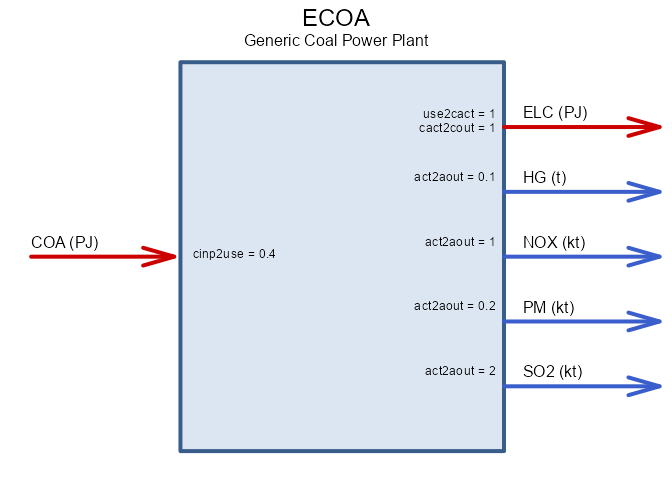Coal-fired power plant.

#### Gas power plant

# Gas-fired power plant ####
set.seed(1e3)
EGAS <- newTechnology(
name = "EGAS",
description = "Generic gas Power Plant",
input = list(
comm = "GAS",
unit = "PJ",
combustion = 1
),
output = list(
comm = "ELC",
unit = "PJ"
),
aux = list(
acomm = c("NOX", "CH4"),
unit = c("kt", "kt")
),
cap2act = 31.536,
ceff = list(
# region = "R1",
# year = c(2015),
comm = c("GAS"),
cinp2use = c(.5)
),
aeff = list(
acomm = c("NOX", "CH4"),
act2aout = c(1.5, .1) # arbitrary
),
afs = list(
slice = "ANNUAL",
afs.up = .8
),
fixom = list(
fixom = 100
),
invcost = list(
invcost = 1200
),
stock = data.frame(
region = c(reg_names, reg_names),
year = c(rep(2015, nreg), rep(2040, nreg)),
stock = c(runif(nreg, .2, 5), rep(0, nreg)) # phasing out
),
start = list(
start = 2010
),
olife = list(
olife = 30
),
slice = "HOUR"
)
draw(EGAS)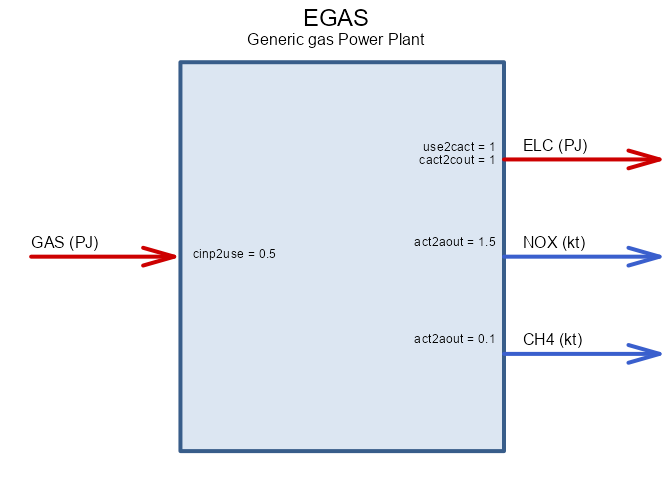Gas-fired power plant.

#### Biomass-to-power plant

# Biomass-cofired power plants ####
EBIO <- newTechnology(
name = "EBIO",
description = "Generic Biomass-fired power plant",
region = reg_names[min(7, nreg)],
input = list(
comm = "BIO",
unit = "PJ",
combustion = 1
),
output = list(
comm = "ELC",
unit = "PJ"
),
aux = list(
acomm = c("NOX", "PM", "CH4"),
unit = c("kt", "kt", "kt")
),
cap2act = 31.536,
ceff = list(
comm = c("BIO"),
cinp2use = c(.3)
),
aeff = list(
acomm = c("NOX", "PM", "CH4"),
act2aout = c(0.1, 1, .01)
),
afs = list(
# activity level bounds per sum of listed slices ('ANNUAL')
slice = "ANNUAL",
afs.up = .5
),
fixom = list(
fixom = 50
),
varom = list(
varom = convert("USD/MWh", "MUSD/PJ", .5) # Assumption
),
invcost = list(
# year = 2015,
invcost = 2000
),
# # stock = data.frame(
#   region = ,
#   year = ,
#   stock = )
# ),
start = list(
start = 2010
),
olife = list(
olife = 30
),
slice = "HOUR"
)
draw(EBIO)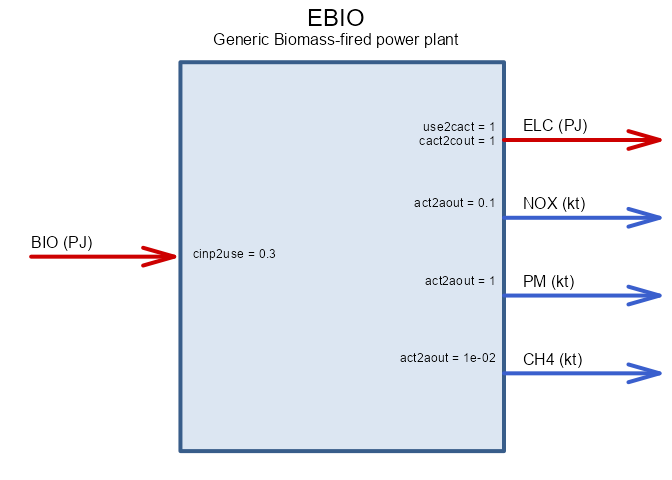Biomass-to-power technology.

#### Nuclear power

# Nuclear power plant #
ENUC <- newTechnology(
name = "ENUC",
description = "Nuclear power plants",
region = reg_names,
input = list(
comm = "NUC",
unit = "PJ"
),
output = list(
comm = "ELC",
unit = "PJ"
),
cap2act = 31.536,
ceff = list(
comm = c("NUC"),
cinp2use = c(.35)
),
af = list(
af.lo = .8
),
fixom = list(
fixom = 30
),
invcost = list(
invcost = convert("USD/kW", "MUSD/GW", 3500)
),
stock = data.frame(
region = reg_names,
year = c(2015, 2060),
stock = 2
),
start = list(
start = 2010
),
end = list(
end = 2030
),
olife = list(
olife = 60
),
slice = "HOUR"
)
draw(ENUC)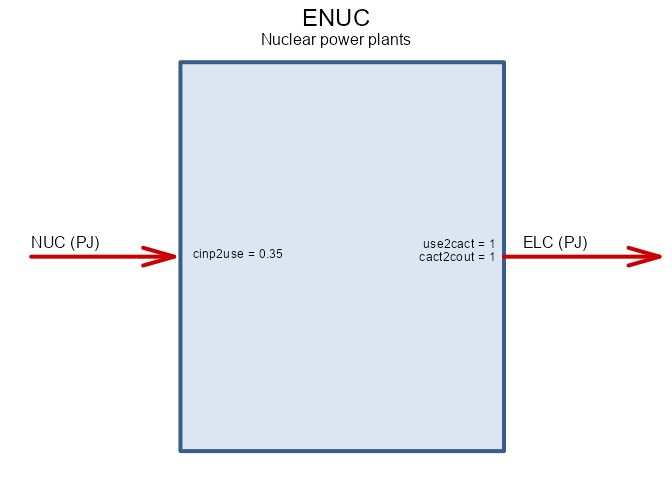Nuclear power technology.

#### Hydro power

EHYD <- newTechnology(
name = "EHYD",
description = "Hydro power plants",
input = list(
comm = "HYD",
unit = "PJ",
combustion = 0
),
region = reg_names[min(3, nreg)],
output = list(
comm = "ELC",
unit = "PJ"
),
cap2act = 31.536,
ceff = list(
comm = c("HYD"),
cinp2use = c(1)
),
af = list(
af.lo = 0.15
),
afs = data.frame(
slice = "ANNUAL",
afs.up = .4,
afs.lo = .3
),
fixom = list(
fixom = 61.4
),
invcost = list(
invcost = 5000
),
stock = data.frame(
region = reg_names[min(3, nreg)],
year = c(2015, 2060),
stock = 5
),
start = list(
start = 2060
),
olife = list(
olife = 80
),
slice = "HOUR"
)
draw(EHYD)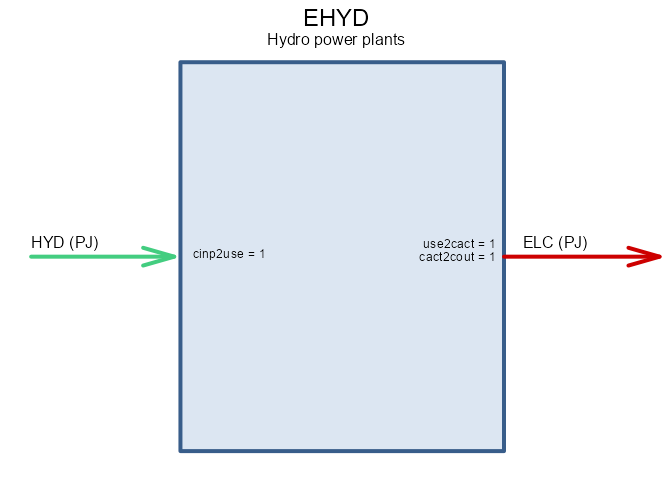Hydro power technology.

#### Weather factors

# The weather information can be obtained from Utopia'a weather agency.
# Here we just randomly generate solar and wind availability factors (AF).
# For wind we can target, say, 25% annual AF, which can be achieved with
# around 35% of the time when the wind is available, and the mean speed of wind
# tending to 80% of maximum output capacity of wind plants.
# For simplicity, let's disregard seasonal components, spatial
# and temporal autocorrelation.
#

# Wind availability function
rwind <- function(n, sh1 = 5, sh2 = 2, pwind = .35) {
# pwind - probability of wind
rbeta(n, sh1, sh2) * sample(0:1, n, TRUE, c(1 - pwind, pwind))
}
# Check
mean(rwind(1e5))

# We can use similar approach for clouds, assuming that expected value for
# sky transparency is 70%, applying it to solar radiation profile by hours.
rclouds <- function(n, sh1 = 3.5, sh2 = 1.5) rbeta(n, sh1, sh2)
mean(rclouds(1e5))
hist(rclouds(1e5), col = "lightblue", probability = T)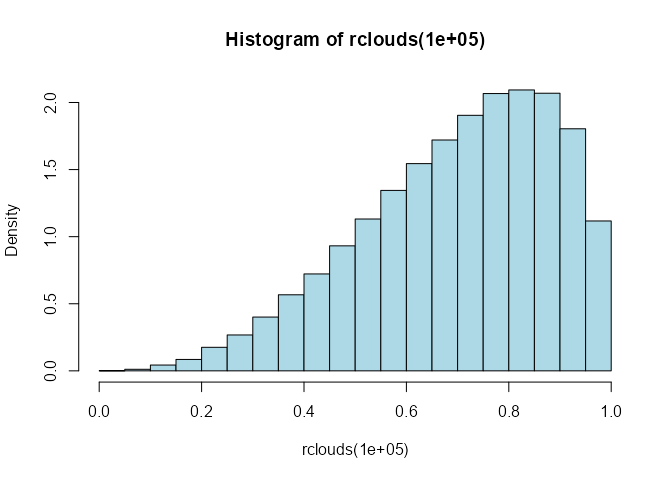# Solar radiation as function of time slice
if (!is.character(slice)) slice <- as.character(slice)
idx <- rep(0, length(slice))
# assuming no difference between months/seasons for sunlight
if_h <- grepl("_h", slice)
if_D <- grepl("_D$", slice) # browser() if (any(if_h)) { # propose function of solar radiation by hours fsun <- function(h) { rad <- -cos(2 * pi * (h) / 23) rad[rad < 0] <- 0 rad } # extract hours as numbers from slices of type "m**_h**" h <- as.integer(substr(slice[if_h], 6, 7)) idx[if_h] <- fsun(h) } else if (any(if_D)) { idx[if_D] <- 1 # assign some sun for Peak hours if_P <- grepl("_P$", slice)
idx[if_P] <- .5
} else {
stop("unknown slices: ", head(paste(slice, collapse = ", ")))
}
idx
}

if (F) { # test
rad <- rbind(slc$slice, solrad(slc$slice))
plot(rad[2, ], type = "l", col = "red")
}

set.seed(1111)
WWIN <- newWeather("WWIN",
description = "Wind availability factor",
slice = "HOUR",
weather = data.frame(
region = rep(reg_names, each = nslc),
year = 2015,
slice = rep(slc$slice, nreg), wval = rwind(nreg * nslc) ) ) WSOL <- newWeather("WSOL", description = "Solar radiation index", slice = "HOUR", weather = data.frame( region = rep(reg_names, each = nslc), year = 2015, slice = rep(slc$slice, nreg),
wval = 0 # assign later
)
)
WSOL@weather$wval <- rclouds(nreg * nslc) * solrad(WSOL@weather$slice)
sum(WSOL@weather$wval) / length(WSOL@weather$wval)

if (F) {
plot(WSOL@weather$wval, type = "l", col = "red") } #### Solar arrays ESOL <- newTechnology( name = "ESOL", description = "Solar PV farm", input = list( comm = "SOL", unit = "PJ" ), output = list( comm = "ELC", unit = "PJ" ), cap2act = 31.536, weather = list( weather = c("WSOL"), waf.up = c(1) # * af.s * WSOL@weather$wval
),
fixom = list(
fixom = 1
),
invcost = list(
invcost = convert("USD/W", "MUSD/GW", 1)
),
stock = data.frame(
region = c(reg_names, reg_names),
year = c(rep(2015, nreg), rep(2035, nreg)),
stock = c(runif(nreg, 0, 3), rep(0, nreg))
),
start = list(
start = 2015
),
olife = list(
olife = 25
)
)
draw(ESOL)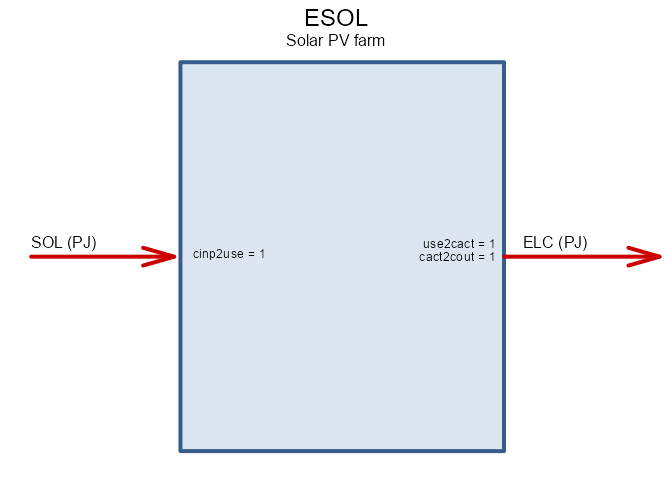#### Wind farms

EWIN <- newTechnology(
name = "EWIN",
description = "Wind power plants",
input = list(
comm = "WIN",
unit = "PJ"
),
output = list(
comm = "ELC",
unit = "PJ"
),
cap2act = 31.536,
ceff = list(
comm = c("WIN"),
cinp2use = 1,
afc.up = 1
),
weather = list(
weather = "WWIN",
comm = "WIN",
waf.up = 1 #
),
fixom = list(
fixom = 3
),
invcost = list(
invcost = 1500
),
stock = data.frame(
region = c(reg_names, reg_names),
year = c(rep(2015, nreg), rep(2035, nreg)),
stock = c(runif(nreg, 0, 3), rep(0, nreg))
),
start = list(
start = 2015
),
olife = list(
olife = 25
)
)
draw(EWIN)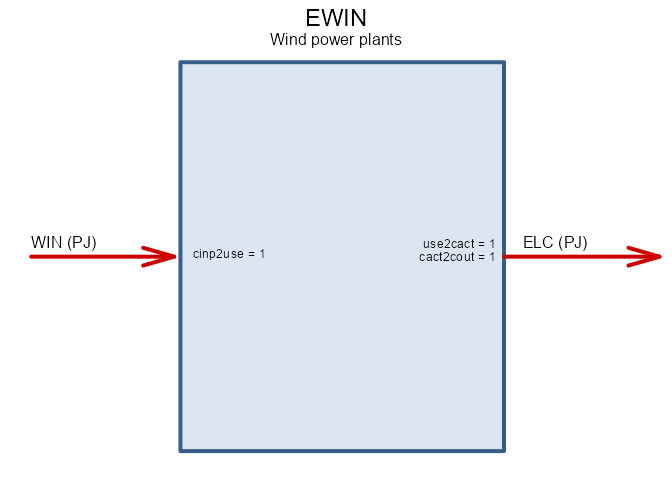#### Energy storage systems


STGELC <- newStorage(
name = "STGELC",
commodity = "ELC",
description = "Energy storage systems",
cap2stg = 1,
olife = list(olife = 10),
invcost = list(
invcost = convert("USD/kWh", "MUSD/PJ", 200)
),
seff = data.frame(
stgeff = .99,
inpeff = .9,
outeff = .95
)
)

The trade is basically a definition of trade routes between regions for each commodity and each direction. There are many ways to arrange the trade routes, which is growing with the number of regions. Here we define trade between neihbor regions, which opens flows between all uninsulated regions. The neighbor regions can be obtained based on the GIS info.

# Neighbor regions
nbr <- spdep::poly2nb(gis, snap = .001)
names(nbr) <- gis@data$region # Trade matrix trd_nbr <- matrix(rep(NA, nreg * nreg), nrow = nreg, dimnames = list(reg_names, reg_names) ) for (i in 1:length(reg_names)) { trd_nbr[i, nbr[[i]]] <- 1 trd_nbr[nbr[[i]], i] <- 1 } dim(trd_nbr) head(trd_nbr) Trade routes matrix. R1 R2 R3 R4 R5 R6 R7 R1 NA 1 1 NA NA NA NA R2 1 NA 1 1 NA NA NA R3 1 1 NA 1 NA NA 1 R4 NA 1 1 NA 1 NA NA R5 NA NA NA 1 NA NA NA R6 NA NA NA NA NA NA 1 R7 NA NA 1 NA NA 1 NA We can visualize the open trade routes over the map. # Data frame of trade routes trd_dt <- as.data.frame.table(trd_nbr, stringsAsFactors = F) trd_dt <- trd_dt[!is.na(trd_dt$Freq), ] # drop NAs
dim(trd_dt)
trd_dt <- dplyr::distinct(trd_dt) # drop duplicates
dim(trd_dt)
names(trd_dt) <- c("src", "dst", "trd")

trd_rou <- left_join(trd_dt, reg_centers[, 1:3], by = c("src" = "region"))
trd_rou <- left_join(trd_rou, reg_centers[, 1:3], by = c("dst" = "region"))
trd_rou <- trd_rou %>%
rename(
xsrc = x.x, ysrc = y.x,
xdst = x.y, ydst = y.y
)
trd_rou <- as_tibble(trd_rou)

# a <- value
trd_flows_map <-
ggplot(data = ggis) +
geom_polygon(aes(x = long, y = lat, group = group),
fill = "wheat",
colour = "white", alpha = 1, size = .5
) + # aes fill = id,
coord_fixed(1.) +
guides(fill = FALSE) + # do this to leave off the color legend
theme_void() +
labs(title = "Open interregional electricity trade routes") +
theme(
plot.title = element_text(hjust = 0.5),
plot.subtitle = element_text(hjust = 0.5)
) +
geom_segment(aes(x = xsrc, y = ysrc, xend = xdst, yend = ydst),
data = trd_rou, inherit.aes = FALSE, size = 5,
alpha = 1, colour = "dodgerblue", lineend = "round", show.legend = T
) +
geom_point(data = reg_centers, aes(x, y), colour = "red") +
geom_segment(aes(x = xsrc, y = ysrc, xend = xdst, yend = ydst),
data = trd_rou, inherit.aes = FALSE, size = .1,
arrow = arrow(
type = "closed", angle = 15,
length = unit(0.15, "inches")
),
colour = "white", alpha = 0.75,
lineend = "butt", linejoin = "mitre", show.legend = T
) # , name = "Trade, PJ"
trd_flows_map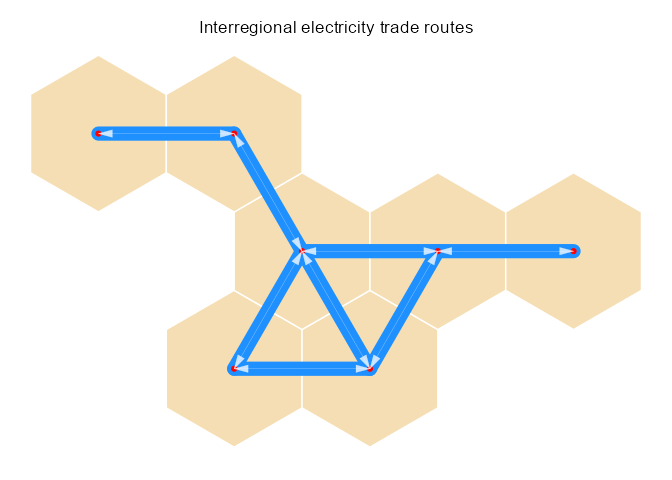For every trade-route, direction, and every traded commodity we have to define an object of class trade to open the trades in the model. To simplify the process, we can group the trades by source regions. Therefore we will have up to 7 trade objects.

For electric power sector model, we can potentially consider trades as dispatch, adding various levels of voltages, or we may want to add an option for primary commodities trade between regions. In this simplified demonstration we define trade for electricity itself, without consideration of different levels of voltages, as well as investments in grid. It can be done adding into the model more technologies, such as transformers, and the grid-lines. Though this type of models don’t have such details as grid simulation models have. …

# Define trade losses
trd_dt$distance_km <- 0. for (i in 1:dim(trd_dt)) { rg_dst <- trd_dt$dst[i]
rg_src <- trd_dt$src[i] # lab_dst <- reg_centers[rg_dst, 2:3] # lab_src <- reg_centers[rg_src, 2:3] lab_dst <- reg_centers[reg_centers$region == rg_dst, c("x", "y")]
lab_src <- reg_centers[reg_centers$region == rg_src, c("x", "y")] trd_dt$distance_km[i] <- raster::pointDistance(
lab_src, lab_dst, T
) / 1e3
}

# Assume losses 1% per 1000 km
trd_dt$losses <- round(trd_dt$distance_km / 1e3 * 0.01, 4)
trd_dt$teff <- 1 - trd_dt$losses
# Assuming 0.1 US cents per kWh per 1000 km for the transmission
trd_dt$cost <- round(trd_dt$distance_km / 1e3 *
convert("USD/kWh", "MUSD/GWh", .001), 5)
trd_dt <- as_tibble(trd_dt)

# A repository for trades, related technologies and commodities
TRD_ELC_ALL <- newRepository(name = "TRD_ELC_ALL")
# Define trade object for each route
for (src_nm in unique(trd_dt$src)) { # The loop creates trade-objects for every region with neighbors, ii <- trd_dt$src %in% src_nm # select routes for the source region
dst_nms <- trd_dt$dst[ii] # all destinations from the region trd_nm <- paste0("TRD_ELC_", src_nm) # Trade object name cmd_nm <- "ELC" cmd_nm_nbr <- "ELC" # Trade class for every route trd <- newTrade(trd_nm, commodity = cmd_nm, source = src_nm, destination = dst_nms, trade = data.frame( src = src_nm, dst = dst_nms, ava.up = convert("GWh", "PJ", 100), # Maximum capacity per route in GW teff = trd_dt$teff[ii], # trade losses
cost = trd_dt$cost[ii] # trade costs # markup = trd_dt$cost[ii] # and/or markup
),
# !!! New stuff - testing
capacityVariable = TRUE, # endogenous trade capacity & investments
# bidirectional = TRUE, # oppenes reverse direction of the trade
invcost = data.frame(
region = src_nm,
# dst = dst_nms,
invcost = trd_dt$distance_km[ii] / 1e3 * 250 # Assuming$250 MUSD/1000km
)
)
}

### Bi-directional trade routes with endogenous capacity - !!!testing
# first - find unique pairs of regions
trd_dt2 <- trd_dt
trd_dt2$src <- trd_dt$dst
trd_dt2$dst <- trd_dt$src
trd_dt3 <- bind_rows(trd_dt, trd_dt2)
trd_dt <- unique(trd_dt3)
trd_dt <- trd_dt[order(trd_dt$src), ] rm(trd_dt2, trd_dt3) TRBD_ELC_ALL <- newRepository(name = "TRBD_ELC_ALL") for (i in 1:dim(trd_dt)) { src <- trd_dt$src[i]
dst <- trd_dt$dst[i] trd_nm <- paste0("TRBD_ELC_", src, "_", dst) # Trade object name cmd_nm <- "ELC" # Trade class for every route trd <- newTrade(trd_nm, commodity = cmd_nm, source = c(src, dst), destination = c(src, dst), trade = data.frame( src = src, dst = dst, ava.up = convert("GWh", "PJ", 1000), # Maximum capacity per route in GW teff = trd_dt$teff[i] # trade losses
# cost = trd_dt$cost[i] # trade costs # markup = trd_dt$cost[ii] # and/or markup
),
# !!! New stuff - testing
capacityVariable = TRUE, # The trade route has capacity (not just flow) and can be endogenous
# bidirectional = TRUE, #
invcost = data.frame(
region = src,
# dst = dst,
year = 2010,
invcost = trd_dt$distance_km[i] / 1e3 * 250 # Assuming$250 MUSD/1000km
),
# olife = data.frame(
olife = 80,
# ),
cap2act = convert("GWh", "PJ", 24 * 365)
)

}

names(TRBD_ELC_ALL@data)
TRBD_ELC_ALL@data[]

COAIMP <- newImport(
name = "COAIMP",
description = "Coal import from ROW",
commodity = "COA",
imp = list(
# Let's make it a bit more expensive than domestic coal
year = SUP_COA@availability$year, price = SUP_COA@availability$cost * 1.2
)
)

GASIMP <- newImport(
name = "GASIMP",
description = "Natural gas import from ROW",
commodity = "GAS",
imp = list(
# Let's make it a bit more expensive than domestic coal
year = SUP_GAS@availability$year, price = SUP_GAS@availability$cost * 1.2
)
)

# OILIMP <- newImport(
#   name = "OILIMP",
#   description = "Oil import from ROW",
#   commodity = "OIL",
#   imp = list(
#     # Let's make it a bit more expensive than domestic coal
#     year = SUP_OIL@availability$year, # price = SUP_OIL@availability$cost * 1.2
#   )
# )

### The model

reps <- add(
newRepository("utopia_repository"),
# Commodities
ELC, # electricity
COA, GAS, NUC, HYD, BIO, # energy
WIN, SOL, # renewables
CO2, PM, NOX, SO2, HG, CH4, # emissions
# Supply
SUP_NUC, SUP_COA, SUP_GAS, RES_HYD,
RES_SOL, RES_WIN,
# Import from ROW
COAIMP, GASIMP,
# Technologies
ECOA, EGAS, ENUC, EHYD,
# EWIN, ESOL,
# TRD_ELC_ALL, # repository with electricity trade routes
DEM_ELC # ELC demand with load curve (24 hours x 12 months)
)

length(reps@data)
names(reps@data)

mdl <- newModel("UTOPIA",
debug = data.frame( # comm = "ELC",
dummyImport = 1e6,
dummyExport = 1e6
),
region = reg_names,
discount = 0.05,
slice = timeslices,
# slice = unlist(timeslices2),
repository = reps,
# GIS = gis,
early.retirement = F
)

We can check the shares of the time-slices which are auto-calculated assuming that all slices have the same weights. This works for 24 hours, but the weights for every month can be specified to take the difference in months length.

ANNUAL MONTH HOUR share
ANNUAL W D 0.0937500
ANNUAL W N 0.1250000
ANNUAL W P 0.0312500
ANNUAL R D 0.1145833
ANNUAL R N 0.1145833
ANNUAL R P 0.0208333
ANNUAL S D 0.1250000
ANNUAL S N 0.0937500
ANNUAL S P 0.0312500
ANNUAL A D 0.1145833
ANNUAL A N 0.1145833
ANNUAL A P 0.0208333

#### The model horizon and annual time-steps (milesone years)

If we don’t need year-by-year steps, milestone years (MSY) can be specifyed to reduce the model dimention. The function setMilestoneYears takes as arguments the model start year and the intervals between MSYs, and calculates the interval years (start and end) for each milestone year.

mdl <- setMilestoneYears(mdl, start = 2015, interval = c(1, 2, rep(5, 8)))
mdl@sysInfo@milestone

## Scenarios


name = "GRID",
# tmp.path = "C:/solwork",
solver = mysolver,
tmp.name = "UTOPIA_GRID",
tmp.del = F,
trim = T
)
summary(scen_GRID)


scen_RBAU <- solve(add(mdl, TRBD_ELC_ALL, WWIN, WSOL, EWIN, ESOL, STGELC), #
name = "RBAU",
# tmp.path = "C:/solwork",
solver = mysolver,
# solver = "GLPK",
# solver = "GAMS",
tmp.name = "UTOPIA_REN",
tmp.del = F
)
summary(scen_RBAU)

## Quick look at the results

# scen <- scen_BASE
scen <- scen_RBAU

# Quick check
scen@modOut@variables$vObjective summary(scen) ### getData function sns <- list(scen_BASE, scen_GRID, scen_RBAU) getData(sns, name = "vObjective", merge = T) getData(scen_BASE, name = "vTechOutTot", comm = "ELC", merge = F) getData(sns, name = "vTechOutTot", comm = "ELC", merge = F) ### Pivot tables # getData format pivot(sns, comm = "ELC", name = "vTechOut") ### Some figures elc_out <- getData(sns, name = "vTechOutTot", comm = "ELC", merge = T) elc_out elc_out <- elc_out %>% group_by(comm, year, region, scenario) %>% summarise(GWh = sum(convert("PJ", "GWh", value))) ggplot(elc_out, aes(year, GWh, colour = scenario)) + geom_line(size = 1) + theme_bw() + facet_wrap(. ~ region) ggplot(elc_out, aes(year, GWh, colour = region)) + geom_line(size = 1) + theme_bw() + facet_wrap(. ~ scenario) emis <- getData(sns, name = "vBalance", merge = T, comm = c("NOX", "SO2", "PM", "HG", "CO2") ) %>% group_by(scenario, comm, year) %>% summarise(value = sum(value)) ggplot(emis, aes(year, value, colour = scenario)) + geom_line() + facet_wrap(. ~ comm, scales = "free") # Fuel Mix vTechInp <- getData(sns, name = "vTechInp", tech_ = "^E", merge = T, yearsAsFactors = T) unique(vTechInp$tech)
unique(vTechInp$comm) unique(vTechInp$scenario)

fumx <- vTechInp %>%
group_by(scenario, comm, year) %>%
summarise(PJ = sum(value))
fumx

ggplot(fumx, aes(as.factor(year), PJ, fill = comm)) +
geom_bar(stat = "identity") +
theme_bw() +
labs(x = "Year", fill = "Fuel") +
facet_wrap(. ~ scenario)

# The same by regions
fumxr <- vTechInp %>%
group_by(scenario, region, comm, year) %>%
summarise(PJ = sum(value))

ggplot(fumxr, aes(as.factor(year), PJ, fill = comm)) +
geom_bar(stat = "identity") +
theme_bw() +
labs(x = "Year", fill = "Fuel") +
facet_grid(region ~ scenario)

# Names of all unempty variables and parameters for trade

# Capacity of the trade routes (the Grid)
trd_cap <- getData(scen,
name = "vTradeCap", merge = T,
newNames = c("value" = "GW")
)
trd_cap

trd_cap$src <- sapply(strsplit(trd_cap$trade, "_"), function(x) x)
trd_cap$dst <- sapply(strsplit(trd_cap$trade, "_"), function(x) x)

# Adding location information for regions' centers
trd_cap <- trd_cap %>%
left_join(reg_centers[, 1:3], by = c("src" = "region")) %>%
left_join(reg_centers[, 1:3], by = c("dst" = "region")) %>%
rename(xsrc = x.x, ysrc = y.x, xdst = x.y, ydst = y.y)
# mutate(GWh = convert("PJ", "GWh", value))
trd_cap

ftrd_cap_map <- function(trd_cap, year = sample(unique(trd_cap$year), 1)) { # Function to map grid capacity for a particular year ii <- trd_cap$year == year
# browser()
trd_cap_map <-
ggplot(data = ggis) +
geom_polygon(aes(x = long, y = lat, group = group),
fill = "wheat",
colour = "white", alpha = 1, size = .5
) + # aes fill = id,
coord_fixed(1.) +
guides(fill = FALSE) + # do this to leave off the color legend
theme_void() +
labs(title = paste0("Interregional electricity grid, year = ", year)) +
theme(
plot.title = element_text(hjust = 0.5),
plot.subtitle = element_text(hjust = 0.5)
) +
geom_segment(aes(x = xsrc, y = ysrc, xend = xdst, yend = ydst, size = GW),
data = trd_cap[ii, ], inherit.aes = FALSE,
alpha = 1, colour = "dodgerblue", lineend = "round", show.legend = T
) +
scale_size_area(limits = range(trd_cap$GW), max_size = 8) + geom_point(data = reg_centers, aes(x, y), colour = "red") trd_cap_map } yrs <- sort(unique(trd_cap$year)) # all years
ftrd_cap_map(trd_cap, yrs)
ftrd_cap_map(trd_cap, yrs)
ftrd_cap_map(trd_cap, yrs[length(yrs)])

trd <- getData(scen_RBAU, name = "vTradeIr", comm = "ELC", merge = T)

trd_flo <- trd %>%
left_join(reg_centers[, 1:3], by = c("src" = "region")) %>%
left_join(reg_centers[, 1:3], by = c("dst" = "region")) %>%
rename(xsrc = x.x, ysrc = y.x, xdst = x.y, ydst = y.y) %>%
mutate(GWh = convert("PJ", "GWh", value))

trd_flo
dim(trd_rou)
dim(trd_flo)
summary(trd_flo$GWh) # ss <- unique(trd_flo$slice)

ftrd_flo_map <- function(slice = sample(unique(trd_flo$slice), 1), year = sample(unique(trd_flo$year), 1)) {
# browser()
ii <- trd_flo$slice == slice ii <- ii & trd_flo$year == year
trd_flo_map <-
ggplot(data = ggis) +
geom_polygon(aes(x = long, y = lat, group = group),
fill = "wheat",
colour = "white", alpha = 1, size = .5
) + # aes fill = id,
coord_fixed(1.) +
guides(fill = FALSE) + # do this to leave off the color legend
theme_void() +
labs(title = paste0(
"Interregional electricity trade flows, year = ",
year, ", slice = ", slice
)) +
theme(
plot.title = element_text(hjust = 0.5),
plot.subtitle = element_text(hjust = 0.5)
) +
geom_segment(aes(x = xsrc, y = ysrc, xend = xdst, yend = ydst, size = GWh),
data = trd_flo[ii, ], inherit.aes = FALSE,
alpha = 1, colour = "dodgerblue", lineend = "round", show.legend = T
) +
scale_size_area(limits = range(trd_flo$GWh), max_size = 8) + geom_point(data = reg_centers, aes(x, y), colour = "red") + geom_segment(aes(x = xsrc, y = ysrc, xend = xdst, yend = ydst), data = trd_flo[ii, ], inherit.aes = FALSE, size = .1, arrow = arrow( type = "closed", angle = 15, length = unit(0.15, "inches") ), colour = "white", alpha = 0.75, lineend = "butt", linejoin = "mitre", show.legend = T ) # , name = "Trade, PJ" trd_flo_map } ftrd_flo_map() ftrd_flo_map() if (!exists("homedir")) homedir <- getwd() # This step requires LaTeX installed, as well as 'animation' package # install.packages("animation") library(animation) # A temporary directory for figures figpath <- file.path(homedir, "tmp/fig") if (!dir.exists(figpath)) dir.create(figpath, recursive = T, showWarnings = F) if (!exists("homedir")) homedir <- getwd() setwd(figpath) saveLatex( { n <- 1 for (s in slc$slice) {
pp <- ftrd_flo_map(s, year = 2055)
ggsave(paste0("Rplot", n, ".pdf"), device = "pdf")
n <- n + 1
}
},
use.dev = FALSE,
caption = "Electricity generation and inter-regional trade.",
# label = "blablabla",
ani.dev = "pdf",
ani.type = "pdf",
img.fmt = "Rplot%d.pdf",
ani.width = 8,
ani.height = 10.5,
# documentclass = docclass,
verbose = T
# latex.filename = fname #label = h,
# pdflatex = NULL
# caption = tsdh2datetime(h)
)
setwd(homedir)

figpath2 <- file.path(homedir, "tmp/fig2")
if (!dir.exists(figpath2)) dir.create(figpath2, recursive = T, showWarnings = F)
if (!exists("homedir")) homedir <- getwd()
setwd(figpath2)

# Figures output parameters
scl1 <- 1
dpi <- 160 * scl1
n <- 1
for (y in mdl@sysInfo@milestone$mid) { for (s in slc$slice) {
fname <- paste0("Rplot", n, ".png")
cat(y, " ", s, " ", fname, "\n")
pp <- ftrd_flo_map(s, year = y)
ggsave(fname, pp,
device = "png",
dpi = dpi, width = scl1 * 1920 / dpi, height = scl1 * 1080 / dpi
)
n <- n + 1
}
}

# correct the path below
system("c:/utils/ffmpeg/bin/ffmpeg -r 4 -f image2 -s 1920x1080 -i Rplot%d.png -vcodec libx264 -crf 25 -pix_fmt yuv420p -y utopia_example_trade.mp4")

setwd(homedir)

## Scenarios with constraints

## NXSX: NOX and SO2 control
NOXSO2control <- newConstraint(
"NOXSO2control",
eq = "<=",
rhs = 1e6,
for.each = list(year = NULL),
variable = list(
variable = "vOutTot",
for.sum = list(comm = c("NOX", "SO2"))
)
)

NOXSO2controlMult <- newConstraint(
"NOXSO2control",
eq = "<=",
rhs = 1e6,
for.each = list(year = NULL),
variable = list(
variable = "vOutTot",
for.sum = list(comm = "NOX"),
mult = 1.5
),
# for the case of different multipliers
variable = list(
variable = "vOutTot",
for.sum = list(comm = "SO2"),
mult = 1.25
)
)

NOXSO2controlCum <- newConstraint(
"NOXSO2control",
eq = "<=",
rhs = 1e8,
variable = list(
variable = "vOutTot",
for.sum = list(
comm = c("NOX", "SO2")
)
)
)


GHG50tax <- newTax("GHGtax", comm = "GHG", tax = list(bal = 100))

# GHG50tax <- newTax('HGtax', comm = 'HG', year = 2025:2035, value = data.frame(year = c(2025, 2030), value = c(1, 100)))

)